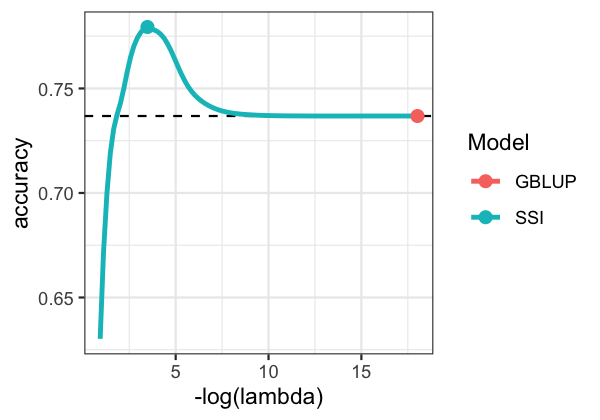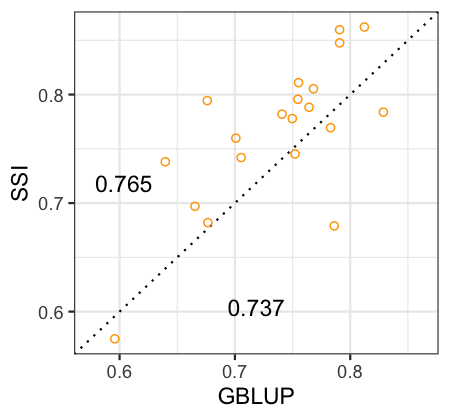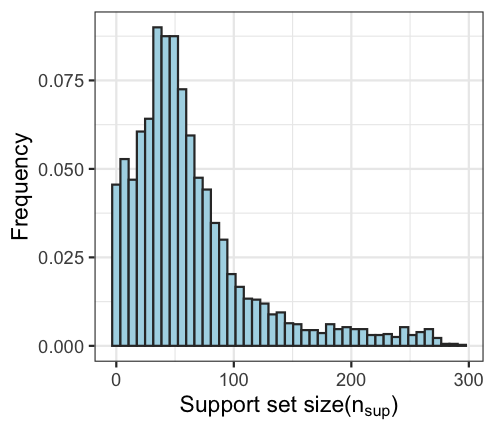# Standard Selection Index

Recall the basic genetic model that decomposes phenotypic observations, $$\boldsymbol{y}=(y_1,…,y_n)'$$, as the sum of breeding values, $$\boldsymbol{u}=(u_1,…,u_n)'$$, and environmental deviations, $$\boldsymbol{\varepsilon}=(\varepsilon_1,…,\varepsilon_n)'$$, as

$\begin{equation*} y_i=u_i+\varepsilon_i \end{equation*}$

Both $$\boldsymbol{u}$$ and $$\boldsymbol{e}$$ are assumed to have null means and $$\text{var}(\boldsymbol{u})=\sigma_u^2 \textbf{G}$$, $$\text{var}(\boldsymbol{\varepsilon})=\sigma_\varepsilon^2 \textbf{I}$$, and $$\text{cov}(\boldsymbol{u},\boldsymbol{\varepsilon}')=\textbf{0}$$, where $$\sigma_u^2$$ and $$\sigma_\varepsilon^2$$ are the common genetic and residual variances, respectively; $$\textbf{G}$$ is the genetic relationship matrix and $$\textbf{I}$$ is an identity matrix.

A standard selection index (SI) predicts the breeding value of an individual ($$u_i$$) using a linear combination of the training phenotypes:

$\begin{equation*} \mathcal{I}_i=\boldsymbol{\beta}'_i\boldsymbol{y} \end{equation*}$

where $$\boldsymbol{\beta}_i=\{\beta_{ij}\}$$ is a vector of weights which are obtained as the solution to the following optimization problem:

$\begin{equation*} \hat{\boldsymbol{\beta}}_i = \underset{\boldsymbol{\beta}_i}{\text{arg min}}\frac{1}{2}\mathbb{E}\left(u_i-\boldsymbol{\beta}'_i\boldsymbol{y}\right)^2 \end{equation*}$

This optimization problem can be reduced to

$\begin{equation*} \hat{\boldsymbol{\beta}}_i = \underset{\boldsymbol{\beta}_i}{\text{arg min}}\left[\frac{1}{2}\boldsymbol{\beta}'_i(\textbf{G}+\theta \textbf{I})\boldsymbol{\beta}_i - \textbf{G}'_i\boldsymbol{\beta}_i\right] \end{equation*}$

where $$\theta=\sigma_\varepsilon^2/\sigma_u^2=(1-h^2)/h^2$$ is the ratio of the residual to the genetic variance, which can be expressed in terms of the heritability, $$h^2$$. The solution to the above problem can be shown to be

$\begin{equation*} \hat{\boldsymbol{\beta}}_i = (\textbf{G}+\theta \textbf{I})^{-1}\textbf{G}_i \end{equation*}$

The vector $$\hat{\boldsymbol{\beta}}_i$$ can be shown to be the $$i^{th}$$ row of the Hat matrix of the BLUPs of the genetic values of the individuals in the prediction set, thus, depending on whether $$\textbf{G}$$ is a pedigree- or genomic-derived relationship matrix, the standard SI is equivalent to a pedigree- (Henderson 1963) or genomic-BLUP, respectively.

# Sparse Selection Index (SSI)

Sparsity (and possibly differential shrinkage on the $$\hat{\boldsymbol{\beta}}_i$$) can be achieved by adding an L1-penalty to the above optimization function; therefore,

$\begin{equation*} \tilde{\boldsymbol{\beta}}_i = \underset{\boldsymbol{\beta}_i}{\text{arg min}}\left[\frac{1}{2}\boldsymbol{\beta}'_i(\textbf{G}+\theta \textbf{I})\boldsymbol{\beta}_i - \textbf{G}'_i\boldsymbol{\beta}_i + \lambda\sum_{j=1}^n|\beta_{ij}|\right] \end{equation*}$

The above optimization problem does not have a closed-form solution; however, solutions can be obtained using a Coordinate Descent algorithm very similar to the one used to solve LASSO problems (Lopez-Cruz et al. 2020). The value $$\lambda=0$$ produces the coefficients of the standard SI (or G-BLUP).

The regularization parameter $$\lambda$$ controls how sparse $$\tilde{\boldsymbol{\beta}}_i$$ will be; this parameter is also expected to affect the accuracy of the index. Therefore, an optimal value of $$\lambda$$ can be found by maximizing the accuracy of the resulting index.

# Genetic analysis

To quantify the prediction accuracy of each of the indices, we randomly divide data set into training (trn) and testing (tst) sets. Predictions were derived by first obtaining $$\hat{\boldsymbol{\beta}}_i$$ for the standard SI and $$\tilde{\boldsymbol{\beta}}_i(\lambda)$$ for the SSI. These coeficicients are obtained using training data, i.e., with $$\textbf{G}=\textbf{G}_{trn}$$ representing the genomic matrix of the training data points, and $$\textbf{G}_i=\textbf{G}_{trn,tst(i)}$$ being the vector containing the genomic relationships between the $$i^{th}$$ data-point of the testing set, with each of the individuals assigned to the training set. This is repeated for each individual in the testing set ($$i=1,...,n_{tst}$$). Subsequently, predictions for each individual are obtained using $$\hat{\mathcal{I}}_i=\hat{\boldsymbol{\beta}}'_i \boldsymbol{y}_{trn}$$ (for the standard SI) and $$\hat{\mathcal{I}}_i(\lambda)=\tilde{\boldsymbol{\beta}}'_i(\lambda) \boldsymbol{y}_{trn}$$ (for the SSI) where $$\boldsymbol{y}_{trn}$$ is a $$n_{trn}\times 1$$ vector with the adjusted-centered phenotypes of the training set.

The implementation of the SI requires heritability estimates. These are derived by fitting a G-BLUP model as above-mentioned within the training set, i.e., using $$\boldsymbol{y}_{trn}$$ and $$\textbf{G}_{trn}$$. We then use the variance parameters estimates to derive $$h^2=\sigma_u^2/(\sigma_u^2 + \sigma_\varepsilon^2 )$$.

Prediction accuracy ($$\rho$$) is measured with the correlation between the phenotype and the index, divided by the square-root of the heritability of the trait, $$\rho=Acc(\mathcal{I})=Cor(\hat{\mathcal{I}}_i,y_i )/h$$ (Dekkers 2007). We apply all methods to the same training-testing partitions and report, from these analyses, the average prediction accuracy and the proportion of times that one method is better than the other.

To determine an optimal value of $$\boldsymbol{\lambda}$$ we implement a calibration analysis using data from the training data only. Specifically, for each training set, we conduct an internal cross-validation (CV) as follows: (i) The training data set is partitioned into $$k$$ subsets. (ii) SSIs are derived over a grid of values of $$\lambda$$ using data from $$k-1$$ folds for training and the data in the $$k^{th}$$ fold as testing. (iii) The resulting curves profiling accuracy ($$\rho$$) by values of $$\lambda$$ are used to identify the value of $$\lambda$$ ($$\hat{\lambda}_{cv}$$) that maximizes accuracy. (iv) Finally, we use all the data from the training set to derive $$\mathcal{I}_i (\hat{\lambda}_{cv})$$ and evaluated the accuracy of the resulting index in the left-out data from the testing set.

# Implementation

To illustrate how to fit Sparse Selection Indices for grain yield, we use data from the Wheat-small data set which is available with the BGLR R-package (Perez and Campos 2014). Sparse Selection Indices will be derived using the SFSI R-package (Lopez-Cruz et al. 2020).

## Data preparation

The following code shows how to prepare data for environment 1; all the analyses hereinafter are based on this data.

library(SFSI)
if(requireNamespace("BGLR")){
data(wheat, package="BGLR")     # Load data from the BGLR package
}

# Select the environment 1 to work with
y <- as.vector(scale(wheat.Y[,1]))

# Calculate G matrix
G <- tcrossprod(scale(wheat.X))/ncol(wheat.X)

# Save data
save(y, G, file="geno_pheno.RData")      

## Heritability and variance components

Implementing the SSI requires an estimate of the heritability. We obtain this using a G-BLUP model $$y_i=\mu+u_i+\varepsilon_i$$ with $$\varepsilon_i \overset{iid}{\sim} N(0,\sigma_\varepsilon^2)$$ and $$\boldsymbol{u}\sim N(\textbf{0},\sigma_u^2 \boldsymbol{G})$$. This model can be fitted with the function fitBLUP included in the SFSI R-package. The BGLR R-package can be also used to fit a Bayesian version of the model. The code below illustrates how to estimate heritability using the function fitBLUP.

load("geno_pheno.RData") # Load data

# Fit model
fm0 <- fitBLUP(y, K=G)
fm0$theta <- fm0$varE/fm0$varU # Residual/genetic variances ratio fm0$h2 <- fm0$varU/(fm0$varU+fm0$varE) # Heritability c(fm0$varU,fm0$varE,fm0$theta,fm0$h2) # Print variance components save(fm0, file="varComps.RData") ## Training-testing partitions The code below produces training (trn, $$70\%$$) and testing (tst, $$30\%$$) partitions. The parameter nPart defines the number of partitions. The output is a matrix with nPart columns containing 1’s and 2’s indexing the observations that are assigned to the training and testing sets, respectively. The object is saved in the file partitions.RData and will be used in later analyses. nPart <- 5 # Number of partitions load("geno_pheno.RData") # Load data nTST <- ceiling(0.3*length(y)) # Number of elements in TST set partitions <- matrix(1,nrow=length(y),ncol=nPart) # Matrix to store partitions seeds <- round(seq(1E3, .Machine$integer.max/10, length=nPart))

for(k in 1:nPart)
{   set.seed(seeds[k])
partitions[sample(1:length(y), nTST),k] <- 2
}
save(partitions, file="partitions.RData")    # Save partitions

## Accuracy of the G-BLUP and of the Sparse SI

The following script shows how to derive SSIs using the partitions above created. The weights of the SSI are computed using the function SSI for nLambda=100 values of $$\lambda$$. The G-BLUP model is fitted for comparison using the function fitBLUP. Estimates of $$\mu$$ and $$h^2$$ are computed internally in the function SSI when these are not provided. These estimates obtained from the G-BLUP model will be passed to the function SSI to save time. Indices denoting training and testing sets are passed through the trn and tst arguments, respectively. The accuracy of the G-BLUP and SSI models are stored in the object accSSI, and saved in the file results_accuracy.RData.

# Load data

accSSI <- mu <- h2 <- c()       # Objects to store results

for(k in 1:ncol(partitions))
{ cat("  partition = ",k,"\n")
trn <- which(partitions[,k] == 1)
tst <- which(partitions[,k] == 2)
yNA <- y;   yNA[tst] <- NA

# G-BLUP model
fm1 <- fitBLUP(yNA, K=G)
mu[k] <- fm1$b # Retrieve mu estimate h2[k] <- fm1$h2       # Retrieve h2 estimate

# Sparse SI
fm2 <- SSI(y,K=G,b=mu[k],h2=h2[k],trn=trn,tst=tst,mc.cores=1,nlambda=100)
fm3 <- summary(fm2)   # Useful function to get results

accuracy <- c(GBLUP=cor(fm1$u[tst],y[tst]), fm3$accuracy)/sqrt(fm0$h2) lambda <- c(min(fm3$lambda),fm3$lambda) df <- c(max(fm3$df),fm3$df) accSSI <- rbind(accSSI,data.frame(rep=k,SSI=names(accuracy),accuracy,lambda,df)) } save(mu,h2,accSSI,file="results_accuracy.RData") ## Displaying Results The following code creates a plot showing the estimated genetic prediction accuracy by values of the penalty parameter (in logarithmic scale). The rightmost point in the plot corresponds to the G-BLUP model (obtained when $$\lambda=0$$). The point at the peak denotes the maximum accuracy (in the testing set) that was obtained by the SSI. load("results_accuracy.RData") dat <- data.frame(do.call(rbind,lapply(split(accSSI,accSSI$SSI),
function(x) apply(x[,-c(1:2)],2,mean))))
dat$Model <- unlist(lapply(strsplit(rownames(dat),"\\."),function(x)x)) dat2 <- do.call(rbind,lapply(split(dat,dat$Mod),function(x)x[which.max(x$acc),])) if(requireNamespace("ggplot2")){ ggplot2::ggplot(dat[dat$df>1,],ggplot2::aes(-log(lambda),accuracy)) +
ggplot2::geom_hline(yintercept=dat["GBLUP",]$accuracy, linetype="dashed") + ggplot2::geom_line(ggplot2::aes(color=Model),size=1.1) + ggplot2::theme_bw() + ggplot2::geom_point(data=dat2,ggplot2::aes(color=Model),size=2.5) }## Cross-validating to obtain an optimal penalization The snippet below can be used to perform, within each trn-tst partition, $$k$$-folds CV to get an ‘optimal’ value of $$\lambda$$ within the training data, and then used to fit an SSI for the testing set. The CV is implemented using the function SSI.CV from the SFSI R-package for one 5-folds CV, this can be set by changing the nCV and nfolds arguments. load("geno_pheno.RData"); load("varComps.RData") load("partitions.RData"); load("results_accuracy.RData") lambdaCV <- accSSI_CV <- dfCV <- c() # Objects to store results for(k in 1:ncol(partitions)) { cat(" partition = ",k,"\n") trn <- which(partitions[,k] == 1) tst <- which(partitions[,k] == 2) # Cross-validating the training set fm1 <- SSI.CV(y,K=G,trn=trn,nlambda=100,mc.cores=1,nfolds=5,nCV=1) lambdaCV[k] <- summary(fm1)$optCOR["lambda"]

# Fit a SSI with the estimated lambda
fm2 <- SSI(y,K=G,b=mu[k],h2=h2[k],trn=trn,tst=tst,lambda=lambdaCV[k])

accSSI_CV[k] <- summary(fm2)$accuracy/sqrt(fm0$h2)
dfCV <- cbind(dfCV, fm2$df) } save(accSSI_CV,lambdaCV,dfCV,file="results_accuracyCV.RData") After running the above analysis, the following snippet can be run to create a plot comparing partition-wise the accuracy of the optimal SSI with that of the G-BLUP. The average accuracies are also shown in the plot. load("results_accuracy.RData"); load("results_accuracyCV.RData") dat <- data.frame(GBLUP=accSSI[accSSI$SSI=="GBLUP",]$acc,SSI=accSSI_CV) rg <- range(dat) tmp <- c(mean(rg),diff(rg)*0.4) if(requireNamespace("ggplot2")){ ggplot2::ggplot(dat,ggplot2::aes(GBLUP,SSI)) + ggplot2::geom_abline(slope=1,linetype="dotted") + ggplot2::theme_bw() + ggplot2::geom_point(shape=21,color="orange") + ggplot2::lims(x=rg,y=rg) + ggplot2::annotate("text",tmp,tmp-tmp,label=round(mean(dat$GBLUP),3)) +
ggplot2::annotate("text",tmp-tmp,tmp,label=round(mean(dat$SSI),3)) }The code below creates a plot showing the distribution of the number of points in the support set for the SSI, across all partitions. load("results_accuracyCV.RData") dat <- data.frame(df=as.vector(dfCV)) bw <- round(diff(range(dat$df))/40)
if(requireNamespace("ggplot2")){
ggplot2::ggplot(data=dat,ggplot2::aes(df,stat(count)/length(dfCV))) +
ggplot2::theme_bw() +
ggplot2::geom_histogram(color="gray20",fill="lightblue",binwidth=bw) +
ggplot2::labs(x=bquote("Support set size(" *n[sup]*")"),y="Frequency")
}## Subject-specific training sets

The next script can be used to create a plot showing (for a single trn-tst partition) the subset of points in the support set, for each individual being predicted. This plot can be made through the function net.plot from the SFSI package.

# Load data
set.size=c(3,1.5,0.2),point.color="gray40",axis.labels=FALSE)